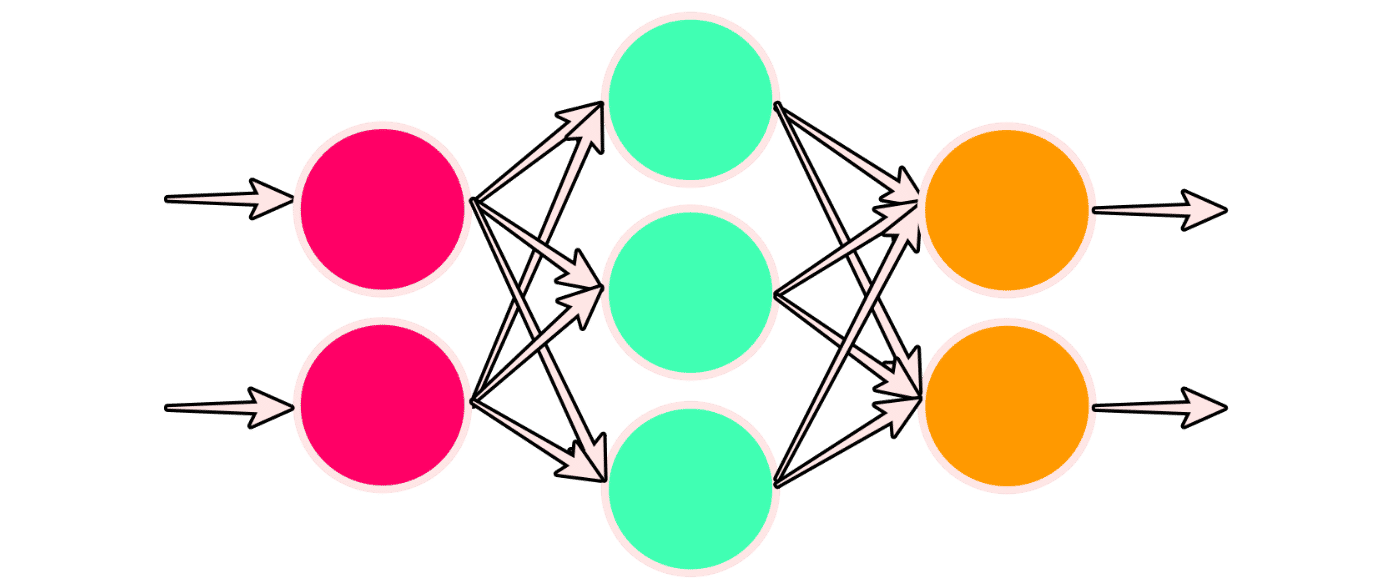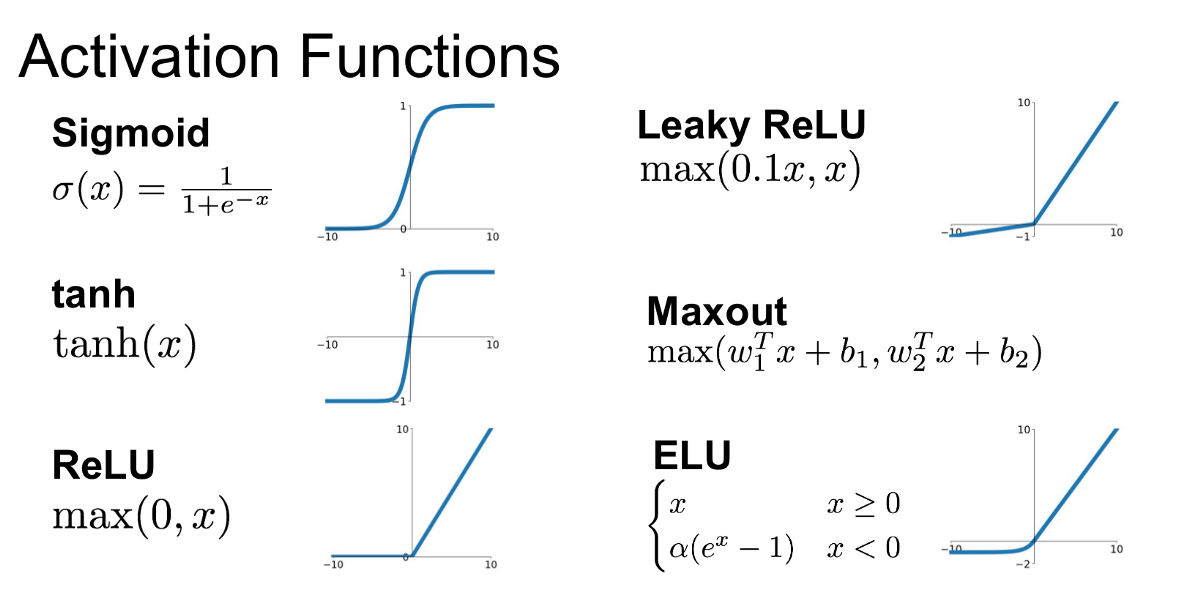# Artificial neural networks

Caution! This article is 3 years old. It may be obsolete or show old techniques. It may also still be relevant, and you may find it useful! So it has been marked as deprecated, just in case.

An artificial neural network is a computing system that is comprised of a collection of connected units called neurons/nodes that are organized into what we call layers.

We have a input layer, several hidden layers, and an output layer. Each node in the input layer of a neural network represents an individual feature of the input data. The number of nodes in the output layer depends on the number of prediction classes present in the training set. The number of nodes in the hidden layer is arbitrary.

The most basic kind of layer is a dense layer, where each output of a layer is computed using every input to the layer, so each node in the a layer is connected to all nodes in the next layer.

Other types of layers are convolutional layers, for image data, recurrent layers, for time series data, etc. They do different transformations to the inputs.Illustration of a neural network with an input layer which has two nodes (in red) one hidden layer (in green) and an output layer with two nodes (in orange).

## Layer weights

Each connection between two nodes has an associated weight, which is just a number. The input will be multiplied by the weight assigned to that connection.

For each node in the second layer, a weighted sum is then computed with each of the incoming connections. This sum is then passed to an activation function.

``````
node output = activation ( weighted sum of inputs )
= activation ( a1w1 + a2w2 + ... + aNwN )
``````

Forward pass: Once we obtain the output for a given node, it is passed as input to the nodes in the next layer. The weights are updated as the net learns.

## Activation function

In an artificial neural network, an activation function is a non-linear function that does some transformation on the weighted sum of a node's inputs. The result activates or deactivates the neuron / node. Pretty much like brain neurons work.A Sigmoid activation function transforms negative or positive inputs into an ouput between zero and one. A ReLU (Rectified Linear Unit) cuts all values less than or equal to zero.

If we only had linear transformations of our data values during a forward pass, the learned mapping in our network from input to output would also be linear. Having non-linear activation functions allows our neural networks to compute arbitrarily complex functions.